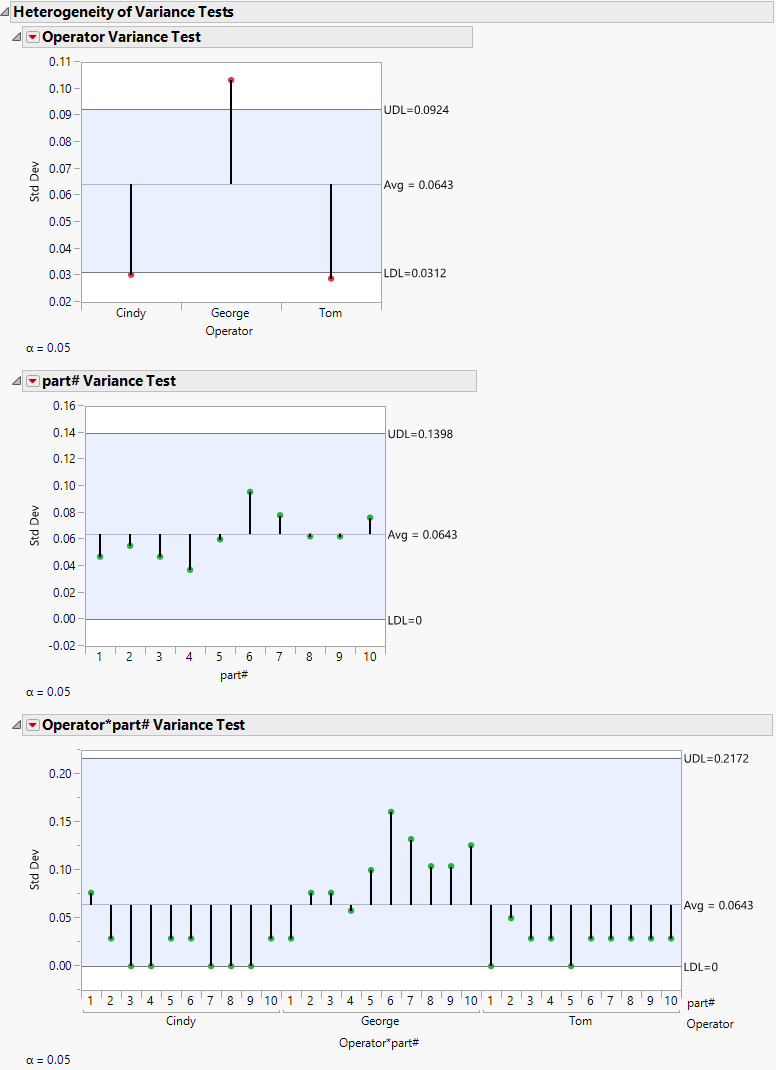For the latest version of JMP Help, visit JMP.com/help.

Publication date: 11/29/2021

## Example of the Heterogeneity of Variance Test

Suppose that you have data containing part measurements. Three operators (Cindy, George, and Tom) each took measurements of 10 parts. They measured each part three times, making a total of 90 observations. You want to examine the following:

whether the variance of measurements for each operator are the same or different

whether the variance for each part is the same or different

whether the variance for each Operator*part combination is the same or different

Ideally, you want all of the variances for each of the groups to be considered the same statistically.

1. Select Help > Sample Data Library and open Variability Data/2 Factors Crossed.jmp.

2. Select Analyze > Quality and Process > Variability / Attribute Gauge Chart.

3. Select Measurement and click Y, Response.

4. Select Operator and click X, Grouping.

5. Select part# and click Part, Sample ID.

6. Click OK.

7. Click the Variability Gauge red triangle and select Heterogeneity of Variance Tests.

8. Select Crossed.

9. Click OK.

Figure 5.9 Heterogeneity of Variances Tests ReportNote: Because the method uses simulations, the decision limits can be slightly different each time.

In the Operator Variance test, all three levels exceed the upper and lower decision limits. From this, you conclude that each operator has a different variability from the square root of the average group variance. You might want to examine why the variation between each operator is different.

For the part# Variance test and the interaction (Operator*part#) Variance test, none of the levels exceed the decision limits. From this, you conclude that the variances are not statistically different from the square root of the average group variance. Each part has a similar variance to the other parts, and each Operator*part# combination has similar variance to the other Operator*part# combinations.

Want more information? Have questions? Get answers in the JMP User Community (community.jmp.com).